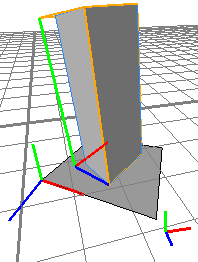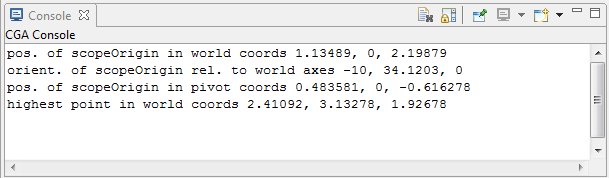# convert function

### Syntax

• float convert(coordinate, fromSystem, toSystem, type, x, y, z)

### Parameters

1. coordinateselector
{ x | y | z }—Coordinate component.
2. fromSystemselector
{ scope | pivot | object | world }—Coordinate system from which to convert.
3. toSystemselector
{ scope | pivot | object | world }—Coordinate system to which to convert.
4. typeselector
{ pos | orient }—Choose interpretation of the (x, y, z) tuple as coordinates or angles.
5. x, y, zfloat
Position (coordinates) / orientation (angles in degrees) in the fromSystem to convert to the toSystem.

### Returns

The selected coordinate component of the tuple (x, y, z), converted from the fromSystem coordinate system to the toSystem coordinate system. The tuple can either describe angles or a position.

### Description

The convert function converts positions and orientations between different coordinate systems.

### Examples

 Using convert() to find the position and orientation of the scope origin in world and pivot coordinates as well as the highest point of the scope in world coordinates ``````Init--> extrude(3) t('0.2, 0, '0.7) s('0.5, '1, '0.5) r(-10, 70, 0) print("pos. of scopeOrigin in world coords " + convert(x, scope, world, pos, 0, 0, 0) + ", " + convert(y, scope, world, pos, 0, 0, 0) + ", " + convert(z, scope, world, pos, 0, 0, 0) ) print("orient. of scopeOrigin rel. to world axes " + convert(x, scope, world, orient, 0, 0, 0) + ", " + convert(y, scope, world, orient, 0, 0, 0) + ", " + convert(z, scope, world, orient, 0, 0, 0) ) print("pos. of scopeOrigin in pivot coords " + convert(x, scope, pivot, pos, 0, 0, 0) + ", " + convert(y, scope, pivot, pos, 0, 0, 0) + ", " + convert(z, scope, pivot, pos, 0, 0, 0) ) print("highest point in world coords " + convert(x, scope, world, pos, scope.sx, scope.sy, scope.sz) + ", " + convert(y, scope, world, pos, scope.sx, scope.sy, scope.sz) + ", " + convert(z, scope, world, pos, scope.sx, scope.sy, scope.sz) )``````The output of the cga code: